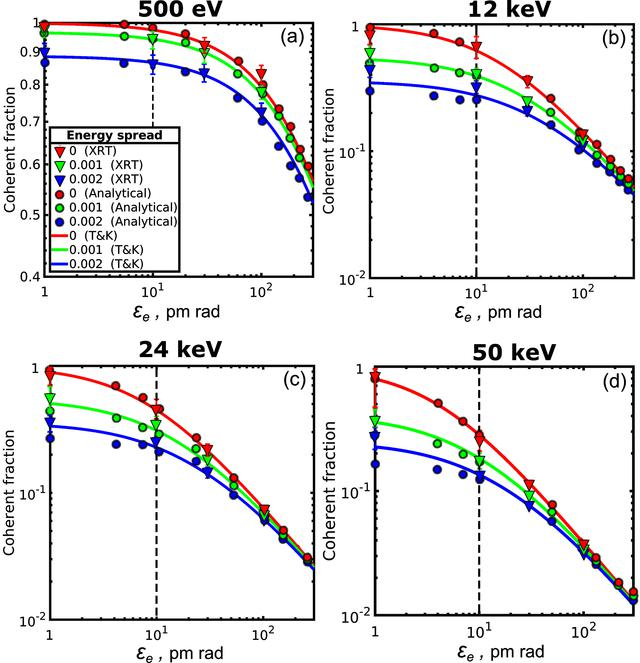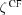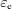disable zoom     view article Figure 2 Coherent fraction of radiationas a function of the electron beam emittancefor the different values of the photon energy and energy spread in one transverse direction calculated according to equation (37). Triangles are XRT simulations, circles are analytical calculations, and lines are the values obtained by the Tanaka & Kitamura (2009) (T&K) approach [equations (30)–(31)]. Red, green and blue colour correspond to 0, 1 × 10−3 and 2 × 10−3 relative energy spread values, respectively. Note the different scale for 500 eV coherent fraction value. Dashed vertical lines correspond to the value of the electron emittance of 10 pm rad.JOURNAL OFSYNCHROTRONRADIATION
ISSN: 1600-5775
Volume 26| Part 6| November 2019| Pages 1851-1862